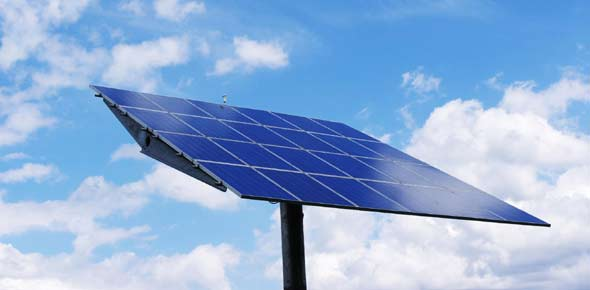# Solar Energy Quiz - Easy

5 Questions | Total Attempts: 835SettingsThis is the quiz which aims to quiz students and give them a challenge. This quiz is the easiest in difficulty.

Related Topics
• 1.
What are solar panels?
• A.

Solar Energy

• B.

A solar cell

• C.

A pack of solar cells

• D.

The sun

• 2.
What are the advantages of solar energy?
1. Can run both in day and night
2. Cheap
3. No carbon emissions
4. High Energy Output
5. The sun is an immense source of energy that can be harnessed with solar energy
• A.

1,2,3

• B.

3,5

• C.

1,3,5

• D.

All of the above

• 3.
Where should solar panels be placed?
• A.

Room with fluorescent lighting

• B.

3 storey building beside skyscrapers

• C.

On a large flat-roof factory

• D.

On a car in a multi-storey carpark

• 4.
What is the process of converting solar energy into electricity?
• A.

Solar energy from the sun --> Absorbed by solar cells --> Transferred into homes --> Inverted by grid-tie inverters --> Electricity

• B.

Solar energy from the sun --> Absorbed by solar cells --> Inverted by grid-tie inverters --> Electricity --> Transferred into homes

• C.

Solar energy from the sun --> Inverted by grid-tie inverters --> Absorbed by solar cells --> Electricity --> Transferred into homes

• D.

Solar energy from the sun --> Inverted by grid-tie inverters --> Electricity --> Inverted by grid-tie inverters --> Transferred into homes

• 5.
What are the disadvantages of solar energy?
• A.

High Cost

• B.

Low efficiency

• C.

Requires good location

• D.

All of the above